### 3.1 The Hyperbolic Paraboloid

Revise the properties of the hyperbolic paraboloid! (HYPAR - in Croatian)

#### 3.1.1 Normal curvatures, asymptotic and principal directions

All points of the hyperbolic paraboloid (hypar) are regular. The tangent plane at T cuts a hypar into the pair of rulings. (Hypar is a double ruled surface). The normal line n through T is perpendicular to the tangent plane t T. The pencil of lines (T) in the plane t T contains the tangent lines to all curves on a hypar which pass through a point T.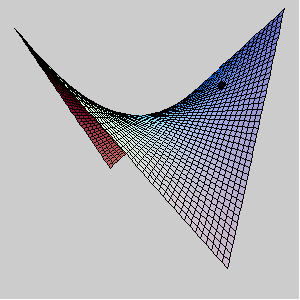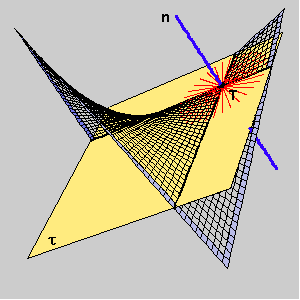We observe the pencil of planes [n] and the curvatures of the normal sections in those planes. The normal curvature of a hypar at a point T is a function which has maximum and minimum at the segment [0,p]. The angles for those extremal values correspond to the principal directions, and the angels where normal curvature is zero correspond to the asymptotic directions.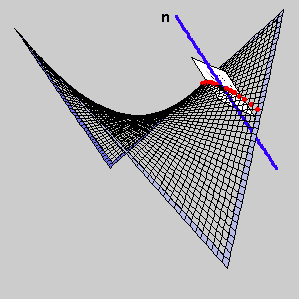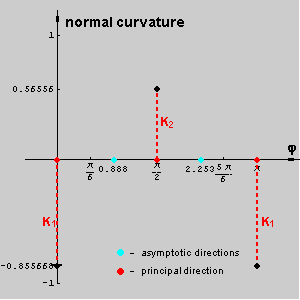The asymptotic directions at a point T lying on a hypar are defined with two rulings through T.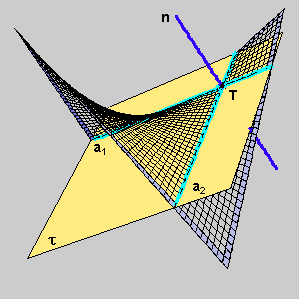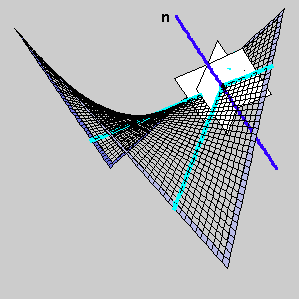The principal directions at a point T lying on a hypar bisect the angle between the asymptotic direction.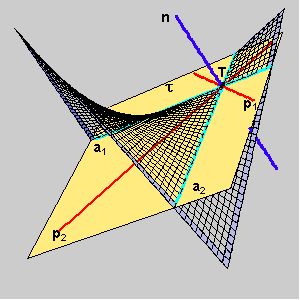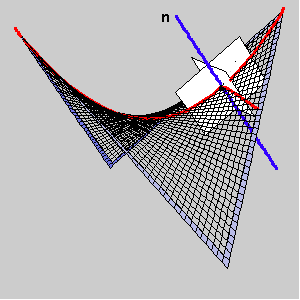The following pictures show the normal sections through the principal and asymptotic directions and a graph of hyper normal curvature at T.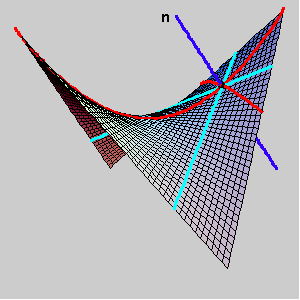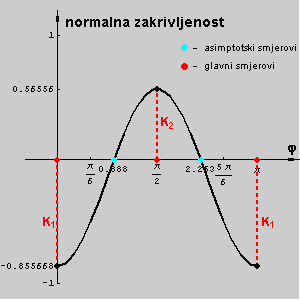#### 3.1.2 Mathematica visualisations of Gaussian and mean curvatures

For the following visualisations we used the periodical Mathematica color function Hue (period 1). It is defined in the following way: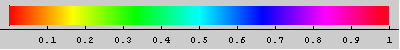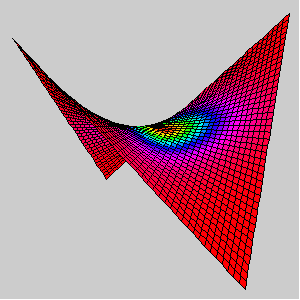A hypar coloured by Hue[G].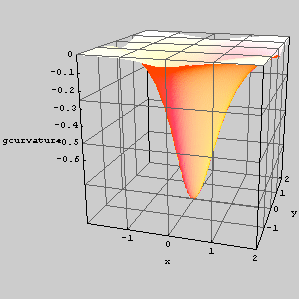The graph of the Gaussian curvature of a hypar.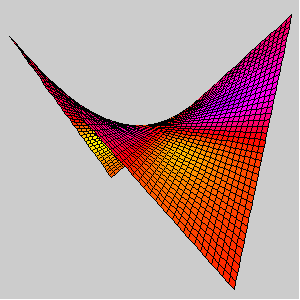A hypar coloured by Hue[H].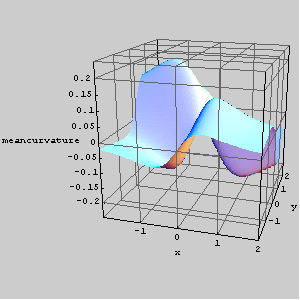The graph of the mean curvature of a hypar.

If you are inerested in Mathematica notebook containing inputs for drawing pictures and animations used in this file download slike_hipar.nb.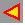back